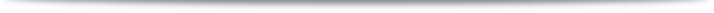﻿ Workshop on Global invariants and Moduli SpacesHome Home > Home
The main theme of this workshop was applications of recent developments for global invairants and geometric invariants to the sutdy of the moduli spaces.
The topics of the workshop include the following:
global aspects of spectral invariants and geometric invariants over moduli spaces,
geometric understanding of tau function, modular form, KZ equation and Hodge bundle over moduli spaces. The 11 experts gave lectures on these subjects and have discussions on common interests.

Invited Speakers:

Luc Hillairet (Université d'Orléans)
Spectral variations of translation surfaces.

Takashi Ichikawa (Saga University)
Modular forms and invariants for algebraic curves.

Alexey Kokotov (Concordia University)
Dmitry Korotkin (Concordia University)
: Baker-Ahhiezer spinor kernel and tau-function on moduli spaces of meromorphic differentials.

Andrew McIntyre (Bennington College)
Chern-Simons invariants, determinant of Laplacian, and tau functions

Shu Oi (Waseda University)
The KZ equation on the moduli space \$mathcal{M}_{0,n}\$ and the Riemann-Hilbert problem II

Yuji Terashima (Tokyo Institute for Technology)
Torsion functions on moduli spaces

Kimio Ueno (Waseda University)
The KZ equation on the moduli space \$mathcal{M}_{0,n}\$ and the Riemann-Hilbert problem, I

Lin Weng (Kyushu University)
General Uniformity of Zeta Functions

Richard Wentworth (University of Maryland)
Gluing formulas for determinants of Dolbeault laplacians on Riemann surfaces

Anton Zorich (University of Paris 7)
: Lyapunov exponents of the Hodge bundle (joint work with A. Eskin and M. Kontsevich)

Organizer:
Jinsung Park(KIAS)

Contact:
Kangwon Lee (